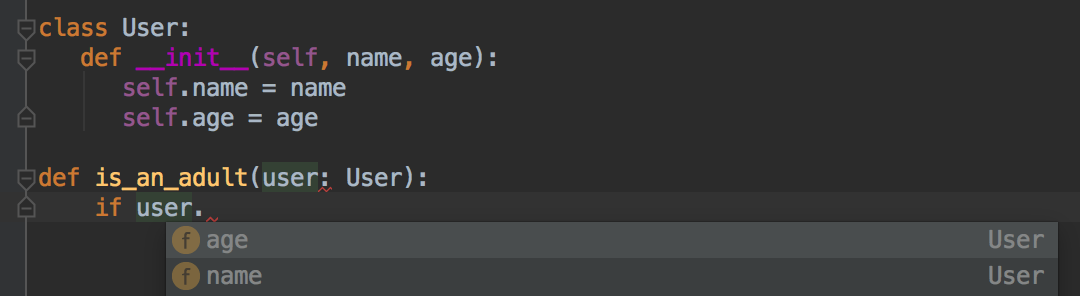# Data classes in Python

Posté le Sat 27 October 2018 dans coding • 5 min de lecture

Cet article est le numéro 3 de la série "type systems" :

Data classes are a brand-new feature in Python 3.7 (PEP 557). They have a lot of benefits since they are a way to:

• declare a type for pieces of data, enabling IDEs autocomplete and type checking features;
• reduce the code size by using a concise syntax for classes definitions.

These two points are demonstrated throughout this blog post.

## Data classes as data types

Data can be represented by several ways:

• For atomic elements, we can use basic types such as int, float or str.
• For more advanced pieces of data, we can use containers, such as list or dict. Containers can store basic types and also be nested, allowing complex data representations.

The container types are however very generic since a dict does not impose restrictions on the keys it stores. We therefore often use classes to store data. It enables a usefull autocomplete feature provided by IDEs. The class name is used to represent the type of the instance of the class.

Let's see a small example of a class in Python 3:

```class User:
def __init__(self, name, age):
self.name = name
self.age = age

bob = User("Bob", 20)
```

bob is an instance of the class User. The same information can be represented by a dict:

```bob = {'name': "Bob",
'age': 20}
```

However, using the former is handy since it enables IDE features:In order to enhance the powers of the IDE, we can add type informations on the fields:

```class User:
def __init__(self, name: str, age: int):
self.name = name
self.age = age
```

Since for storing data, we often set all the arguments of the __init__ method as fields of the object, we may prefer a more concise syntax: data classes. Data classes are a new way to define classes in Python:

```from dataclasses import dataclass

@dataclass
class User:
name: str
age: int
```

It defines automatically the __init__ method. Data classes are regular classes, the only difference being the way to define the class. We will see in the following section that data classes define other usefull methods automatically.

## Data classes as object factories

The drawbacks when definining a class is that you need to define some methods for your objects to behave nicely:

• have a readable and usefull representation of an object,
• compare the equality of two objects in a natural way,
• use objects as keys of a dictionary.

Data classes automatically handle this for you as we will see in this section.

### Have a readable and usefull representation of an object

Two methods are used to have a representation of an object: __repr__ and __str__:

• The __str__ method is called by the built-in function str and therefore by print and format. It should give a readable representation of the object.
• The __repr__ method is called by the buit-in function repr. If possible it should be a valid Python expression enabling to reconstruct the object. It should be unambiguous since it is typically used for debugging.

If bob is a dict, the following code:

```print(bob)
```

outputs:

```{'name': 'Bob', 'age': 20}
```

whereas if bob is an instance of User, it outputs:

```<__main__.User object at 0x105c56780>
```

In order to have a readable output we can define the method __str__ of the class User:

```class User:
def __init__(self, name: str, age: int):
self.name = name
self.age = age

def __str__(self):
return f"User:\nname: {self.name}\nage: {self.age}"
```

With this definition, print(bob) now outputs:

```User:
name: Bob
age: 20
```

It is now as informative as the dict version. However repr(bob) remains unchanged. If we implement __repr__ the following way:

```def __repr__(self):
return f"User(\"{self.name}\", {self.age})"
```

we have a usefull representation of the object. The output of repr is now a Python expression we can evaluate in order to produce the same object:

```bob_copy = eval(repr(bob))
```

With data classes, the __repr__ and __str__ methods are implemented automatically, so both repr(bob) and str(bob) output: "User(name='Bob', age=20)".

### Compare the equality of two objects in a natural way

When using dictionaries, the equality behaves as expected:

```bob = {'name': "Bob", 'age': 20}
alice = {'name': "Alice", 'age': 21}
bob_copy = {'name': "Bob", 'age': 20}

print(bob == bob, bob == alice, bob == bob_copy)
```

outputs : True False True. However it seems unintuitive when using the above defined User class:

```bob = User("Bob", 20)
alice = User("Alice", 21)
bob_copy = User("Bob", 20)

print(bob == bob, bob == alice, bob == bob_copy)
```

outputs True False False without data classes and True False True with data classes. When using data classes, it behaves like the dictionary version. It is because data classes define automatically the __eq__ method.

### Use objects as keys of a dictionary

Say we want store a mapping between users and an other object (e.g. an id in a database). We cannot use dict nor the above User class since it creates an error TypeError: unhashable type.

We can instead use tuples or namedtuples but they have other drawbacks (see Why not just use namedtuple?).

Data classes can generate automatically the __hash__ method when defined as frozen:

```from dataclasses import dataclass

@dataclass(frozen=True)
class User:
name: str
age: int
```

Instances of User can now be used as keys of a dictionary:

```{User("Bob", 20): 1}
```

### Data classes are regular Python classes

Since data classes produce regular Python classes, we can define methods. For instance, we can define the get_older method and the is_an_adult property:

```from dataclasses import dataclass

@dataclass
class User:
name: str
age: int

def get_older(self):
self.age += 1

@property
return self.age >= 18

bob = User("Bob", 17)
bob.get_older()